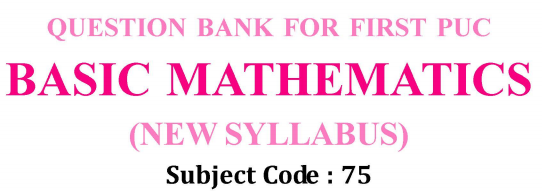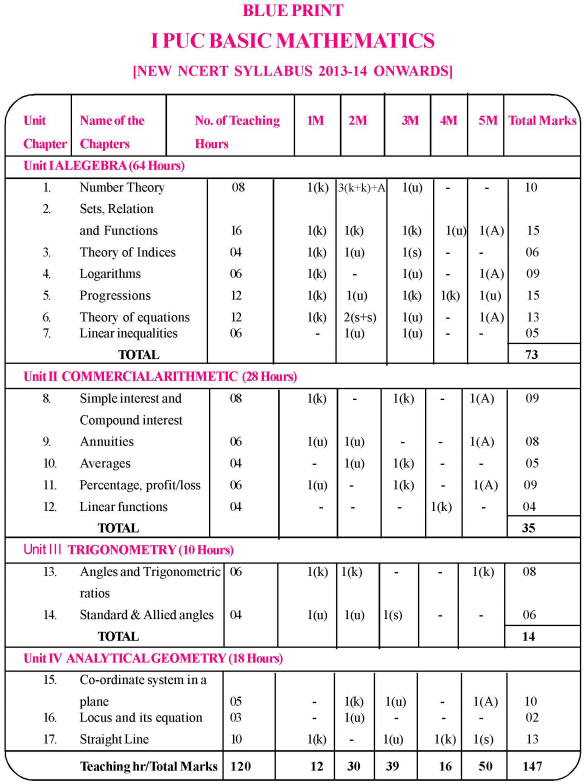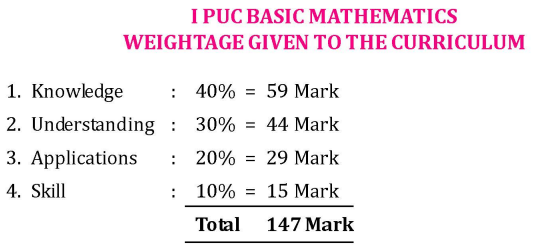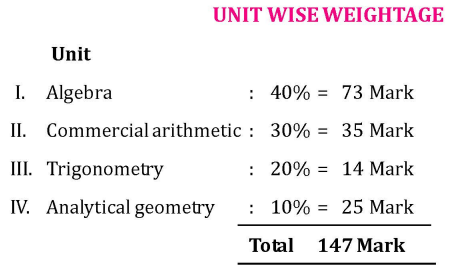# 1st PUC Basic Maths Question Bank with Answers Karnataka

Chapterwise 1st PUC Basic Maths textbook solutions karnataka Board are given here in PDF format for understanding the concepts involved in the syllabus. If you are looking for Chapter-wise KSEEB Solutions for 1st PUC Basic Maths Question Bank with Answers Solutions, Notes, Guide Pdf are given in Chapter wise format then this is the right page for you. Students are advised to download chapter wise 1st PUC Question Bank with Answers Karnataka solutions pdf to take their preparation to the next level. Go through this page and get all chapters pdf links of KSEEB solutions for 1st PUC Basic Maths textbook solutions pdf & learn efficiently to score high marks.

Students can also read 1st PUC Basic Maths Model Question Papers with Answers hope will definitely help for your board exams.## Karnataka 1st PUC Basic Maths Question Bank with Answers

By taking the help of the best resources like Karnataka State Board Solutions for 1st PUC Basic Maths Textbook Questions and Answers you all can easily understand the concepts involved in the latest KTBS Solutions are given in syllabus and gain more subject knowledge. Study 1st PUC Basic Maths Question Bank with Answers Karnataka solutions on a daily basis and be confident to answer all the questions asked in the final exam. Chapter-wise KSEEB solutions for 1st PUC Basic Maths PDF links are available below to download.

### Karnataka 1st PUC Basic Maths Syllabus and Marking Scheme

Karnataka 1st PUC Basic Maths Blue Print of Model Question PaperI PUC Basic Mathematics Weightage Given to the CurriculumI PUC Basic Mathematics Unit Wise WeightageI PUC Basic Mathematics Instructions to Question Paper Setters

1. Part E : 2 marks Question must be from chapters
(i) Number theory (ii) Averages.
2. Part E : 4 marks from chapters
(i) Sets, Relation and Functions
(ii) Progressions
(iii) Linear Functions
(iv) Straight Lines.
3. The question paper consists of five parts A, B, C, D & E.
4. Part A carries 10 marks, Part B carries 20 marks, Part C carries 30 marks, Part D carries 30 marks and Part E carries 10 marks.
5. Write the question numbers properly as indicated in the question paper.

Unit I: Algebra

Chapter 1 Number Theory (Total – 8 hrs)

• 1.0 Introduction
• 1.1 Natural Numbers
• 1.2 Whole numbers
• 1.3 Integers
• 1.4 Odd and Even Numbers
• 1.5 Prime Numbers
• 1.6 Composite Numbers
• 1.7 Fundamental theorem of arithmetic
• 1.8 Least Common Multiple
• 1.9 Highest common factor
• 1.10 Finding H.C.F using the product of numbers given
• 1.11 Finding H.C.F of fractions
• 1.12 Rational Numbers
• 1.13 Irrational Numbers
• 1.14 Real Numbers
• 1.15 Complex numbers

Chapter 2 Sets, Relations and Functions (Total – 16 hrs)

• 2.0 Introduction
• 2.1 Sets
• 2.2 Methods of describing a set
• 2.3 Null set
• 2.4 Singleton set
• 2.5 Finite set and Infinite sets
• 2.6 Equal and equivalent sets
• 2.7 Subset
• 2.8 Universal Set
• 2.9 Operation on Sets
• 2.10 Complement set
• 2.11 Algebra of sets
• 2.12 Venn diagrams
• 2.13 Ordered pairs
• 2.14 Equality of ordered pairs
• 2.15 Cartesian product pairs
• 2.16 Worked examples
• 2.17 Relation
• 2.18 Domain and range of a relation
• 2.19 Inverse relation
• 2.20 Types of relation
• 2.21 Worked Examples
• 2.22 Functions
• 2.23 Domain, co-domain and range
• 2.24 Different types of functions
• 2.25 Worked Examples

Chapter 3 Theory of Indices (Total – 4 hrs)

• 3.1 Introduction
• 3.2 Meaning of an
• 3.3 Laws of Indices

Chapter 4 Logarithms (Total – 6 hrs)

• 4.1 Introduction
• 4.2 Definition of Logarithm
• 4.3 Laws of Logarithm
• 4.4 Common Logarithm

Chapter 5 Progressions (Total – 12 hrs)

• 5.1 Introduction
• 5.2 Sequences
• 5.3 Series
• 5.4 Arithmetic Progressions
• 5.5 nth term of an A.P
• 5.6 Sum to ‘n’ terms of an A.P
• 5.7 Geometric progression
• 5.8 nth term of GP
• 5.9 Sum to n terms of GP
• 5.10 Sum to infinite G.P
• 5.11 Harmonic progression
• 5.12 nth term of H.P
5.13 Arithmetic, Geometric and harmonic means

Chapter 6 Theory of Equations (Total – 12 hrs)

• 6.1 Introduction and definition of equation
• 6.2 Degrees of the equation and different types of equations
• 6.3 Linear equation in one variable
• 6.4 Simultaneous linear equation in two variables and different methods
• 6.5 Quadratic equation and its solution
• 6.6 Nature of the roots of the quadratic equation
• 6.7 Cubic equation, examples and solution
• 6.8 Synthetic division

Chapter 7 Linear Inequalities (Total – 6 hrs)

• 7.1 Introduction
• 7.2 Inequalities
• 7.3 Linear inequalities in one variable
• 7.4 System of linear inequations in one variable
• 7.5 Application of Linear inequalities
• 7.6 Linear inequalities in two variable
• 7.7 System of Linear Inequations in two variables and their graphical solution

Unit II: Commercial Arithmetic

Chapter 8 Simple Interest and Compound Interest (Total – 8 hrs)

• 8.1 Introduction
• 8.2 Simple interest
• 8.3 Compound Interest
• 8.4 Nominal and effective rate of interest
• 8.5 Varying rate of interest
• 8.6 Depreciation
• 8.7 Using compound interest formula for growth rate
• 8.8 Problems related to simple interest and compound interest

Chapter 9 Annuities (Total – 6 hrs)

• 9.1 Definition and types of annuity immediate
• 9.2 Future value of annuity immediate
• 9.3 Present value of annuity immediate
• 9.4 Future value of an annuity due
• 9.5 Present value of an annuity due
• 9.6 Perpetuity
• 9.7 Deferred annuity

Chapter 10 Averages (Total – 4 hrs)

• 10.1 Introduction
• 10.2 Types of Average
• 10.3 Simple Average
• 10.4 Weighted Average
• 10.5 Combined Average

Chapter 11 Percentage, Profit and Loss (Total – 6 hrs)

• 11.1 Conversion of the percentage to ratio fraction, decimal and vice versa
• 11.2 Percentage increase, the percentage decrease
• 11.3 Application problems involving percentages
• 11.4 Profit and Loss, Cost price, Selling price, Profit, Loss, Profit percentage, Loss percentage, definition and formula
• 11.5 Application problems

Chapter 12 Linear Functions (Total – 4 hrs)

• 12.1 Introduction
• 12.2 Definition
• 12.3 Linear Revenue, Cost and profit function
• 12.4 Break-Even Analysis

Unit III: Trigonometry

Chapter 13 Angles and Trigonometric Ratios (Total – 6 hrs)

• 13.1 Introduction
• 13.2 Measurement of angles
• 13.3 Trigonometric ratios of acute angle
• 13.4 Relation between the trigonometric ratios

Chapter 14 Standard Angles and Allied Angles (Total – 6 hrs)

• 14.1 Trigonometric Ratios of standard angles
• 14.2 Signs of Trigonometric ratios
• 14.3 Allied angles

Unit IV: Analytical Geometry

Chapter 15 Co-Ordinate System in a Plane (Total – 5 hrs)

• 15.1 Introduction
• 15.2 Rectangular Cartesian Co-ordinate system in a plane
• 15.3 Distance Formula
• 15.4 Section Formula
• 15.5 Centroid Formula, Midpoint formula
• 15.6 Area of the Triangle and quadrilateral

Chapter 16 Locus and its Equations (Total – 3 hrs)

• 16.1 Introduction
• 16.2 Equation of the locus of a point

Chapter 17 Straight Line (Total – 3 hrs)

• 17.1 Introduction
• 17.2 Slope or Gradient of a line
• 17.3 Slope of Parallel Lines and Perpendicular Lines
• 17.4 SJope of the line joining two points
• 17.5 Standard forms of Equation of straight lines
• 17.6 Equation of a line in general form
• 17.7 Intersection of two lines
• 17.8 Condition for concurrency of three lines
• 17.9 Length of the perpendicular from a point to a line
• 17.10 Distance between parallel lines

We believe that the shared knowledge about Karnataka State Board solutions for 1st PUC Basic Maths Question Bank with Answers benefits you a lot in your exam preparation. If you need more information on the same then stay connected with our site & get updated details on KTBS Solutions PDF for all Subjects.